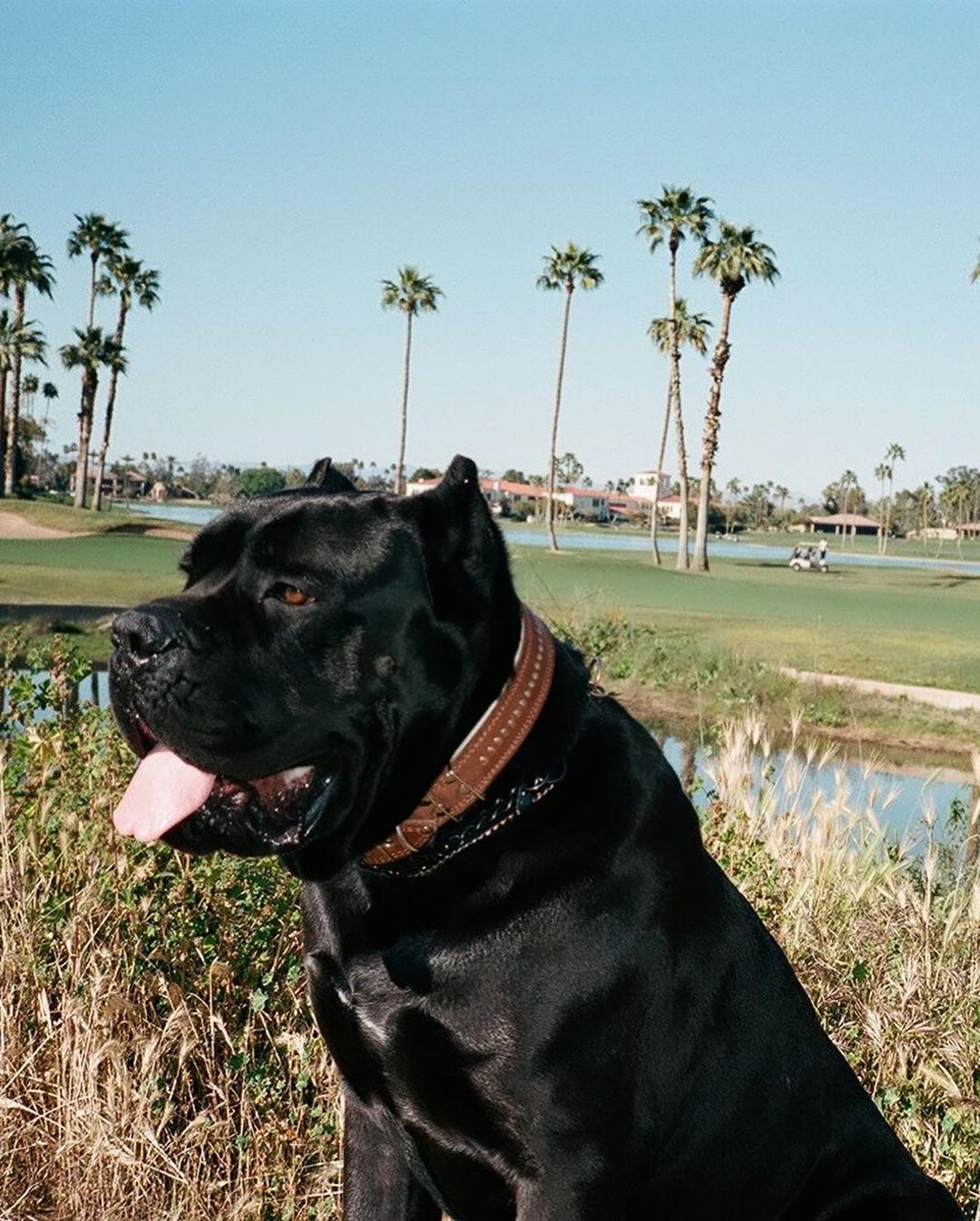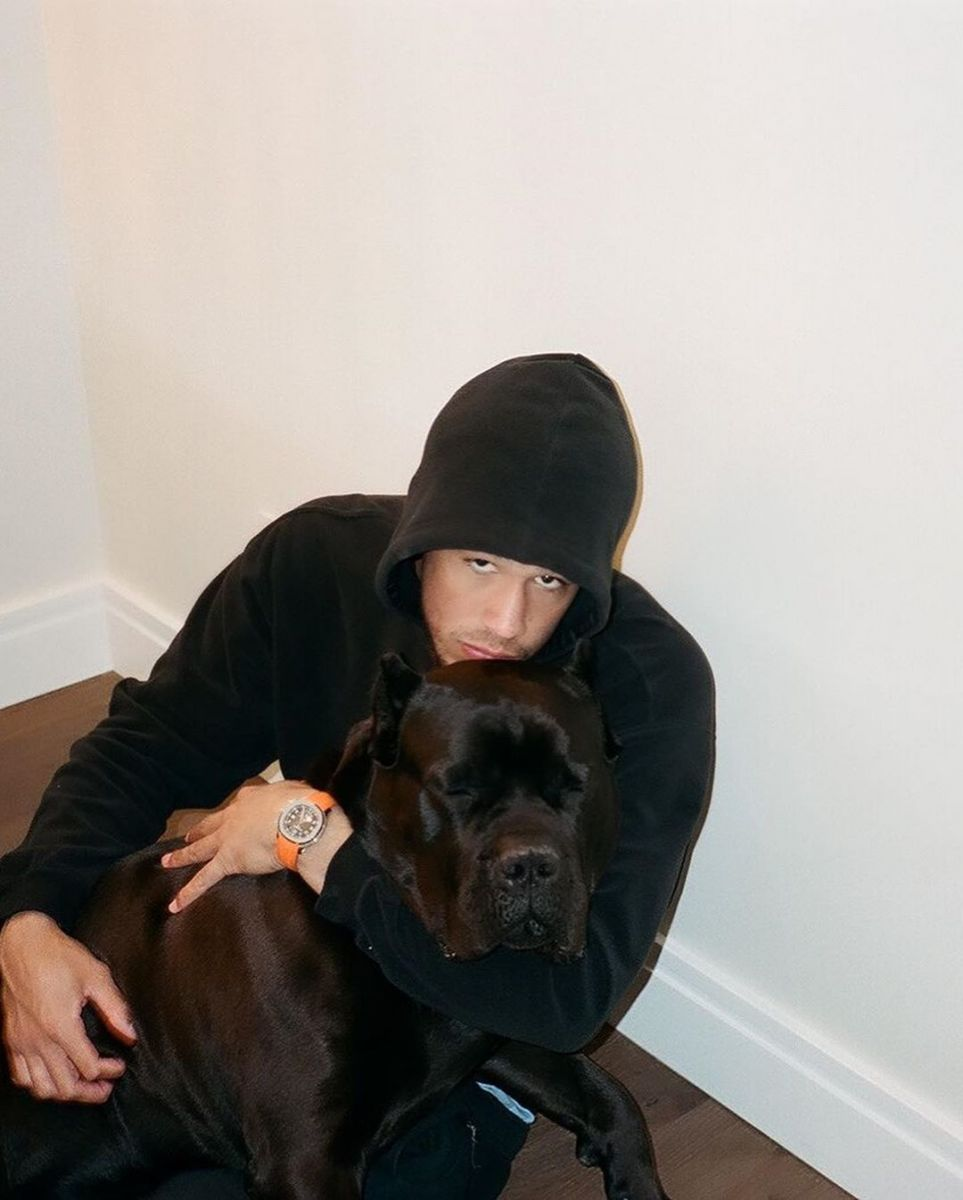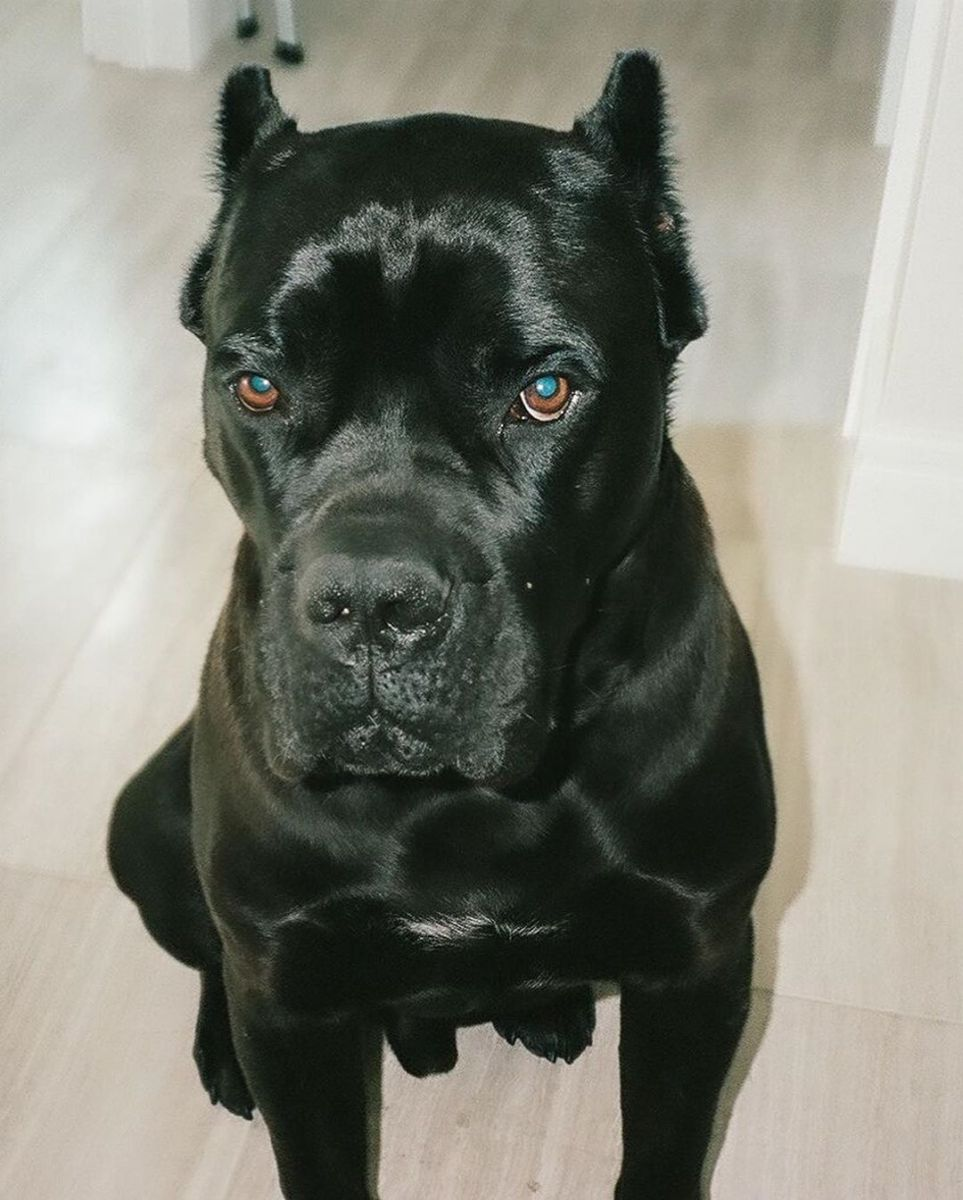# 迷惑行为？布克晒爱犬：祝我首个也是唯一的儿子生日快乐<span style="color: rgb(17, 31, 44); font-family: " microsoft="" yahei",="" "segoe="" ui",="" system-ui,="" roboto,="" "droid="" sans",="" "helvetica="" neue",="" sans-serif,="" tahoma,="" ui="" symbolmyanmar="" text",="" 微软雅黑;="" font-size:="" 14px;="" white-space:="" pre-wrap;="" background-color:="" rgb(255,="" 255,="" 255);"="">(编辑：AphroditeSC30)